# Fracture, mathematical problems of

(diff) ← Older revision | Latest revision (diff) | Newer revision → (diff)

The part of mechanics which studies the stress and strain fields around various defects in solid bodies (cracks, inclusions, dislocations, etc.). Several fracture criteria [a1] are then used to define a dangerous situation which may lead to a fracture of a certain material.

In terms of the mathematical apparatus involved, one may specify two categories of problems: two-dimensional problems and three-dimensional ones. The theory of functions of a complex variable [a3] (cf. also Functions of a complex variable, theory of) and the Fourier transform method [a7] have been used for solving two-dimensional problems (cf. also Two-dimensional problems in fracture mechanics), while three-dimensional problems were treated by the integral-transform method [a4] and the Green's function method [a2] (cf. also Green function).

One may classify within each category problems related to elastic (linear and non-linear), elasto-plastic, visco-elastic bodies, etc. [a1]. The linear elastic two-dimensional theory is the most developed [a3], including the process of crack propagation. Much less is known about three-dimensional problems. A different mathematical treatment is required for infinite, semi-infinite and finite bodies. Exact solutions are known at the moment (1988) only for the case of a flat circular or elliptic crack in an elastic space [a4]. A complete closed form solution was obtained [a2] for a penny-shaped crack in an elastic space, subjected to arbitrary internal tractions.

As an example, consider an elastic space weakened by a flat crack of general shape, subjected to an arbitrary normal traction. The governing integro-differential equation takes the form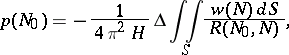(a1)

wheredenotes the crack area,is the two-dimensional Laplace operator,denotes the crack opening displacement,(is the elastic modulus andis the Poisson coefficient), andstands for the distance between two pointsand, with. An exact solution of the equation (a1) is known only for a circle, and in polar coordinates it is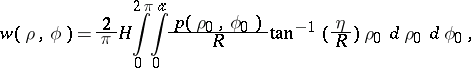(a2)

whereis the radius of the circle and(a3)An approximate solution of (a1) was obtained in [a2] for a crack of general shape subjected to a uniform pressure. Let the crack boundary be defined in polar coordinates as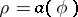. The crack opening displacement is approximated by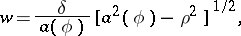(a4)

where the maximum crack displacementis related to the applied pressureby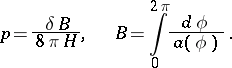(a5)

The coordinate origin is located at the crack centre, which is defined as the point where the value ofattains its minimum. The solution (a4)–(a5) has proven to be fairly accurate for a vast variety of crack shapes [a2].

Consideration of a general flat crack subjected to a shear loadingleads to the governing integro-differential equation [a2]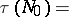(a6)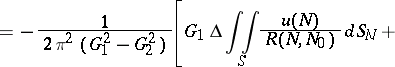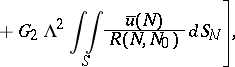where the complex tangential displacement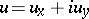was introduced, and(a7)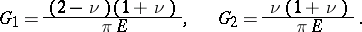An exact closed form solution of (a6) was obtained recently for a circle, and is [a2]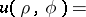(a8)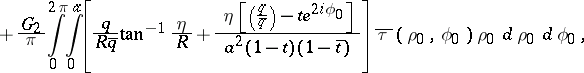whereandare defined by (a3), an overbar indicates the complex conjugate value, and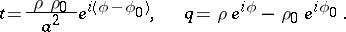An approximate solution can be derived for a general flat crack subjected to a polynomial shear loading [a2]. In the simplest case of a uniform shear, the crack face displacements are approximated by the expression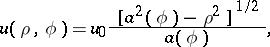(a9)

where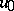is related to the applied loadingby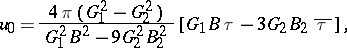(a10)

withdefined by (a5) and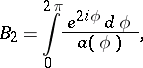Formula (a10) is exact for an ellipse and is expected to perform fairly accurately for various crack shapes.

The stress intensity factors (SIF) are very important parameters in fracture mechanics. They are defined as follows: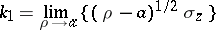for the stress intensity factor of mode I, and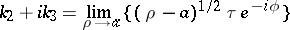for the modes II and III loadings. In the case of a penny-shaped crack, one can write for an arbitrary distributed pressure [a1]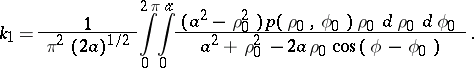In the general case of arbitrarily distributed shear loading, the stress intensity factors take the form [a2]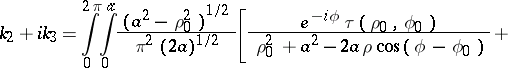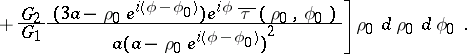There is no general expression for the stress intensity factor for any shape other than circular. Some particular cases of loading of an elliptical crack were considered in [a4]. Various three-dimensional problems of thin inclusions are solved in [a5]. The mathematical description of crack propagation is still in the process of development.

How to Cite This Entry:
Fracture, mathematical problems of. Encyclopedia of Mathematics. URL: http://encyclopediaofmath.org/index.php?title=Fracture,_mathematical_problems_of&oldid=18371
This article was adapted from an original article by V.I. Fabrikant (originator), which appeared in Encyclopedia of Mathematics - ISBN 1402006098. See original article